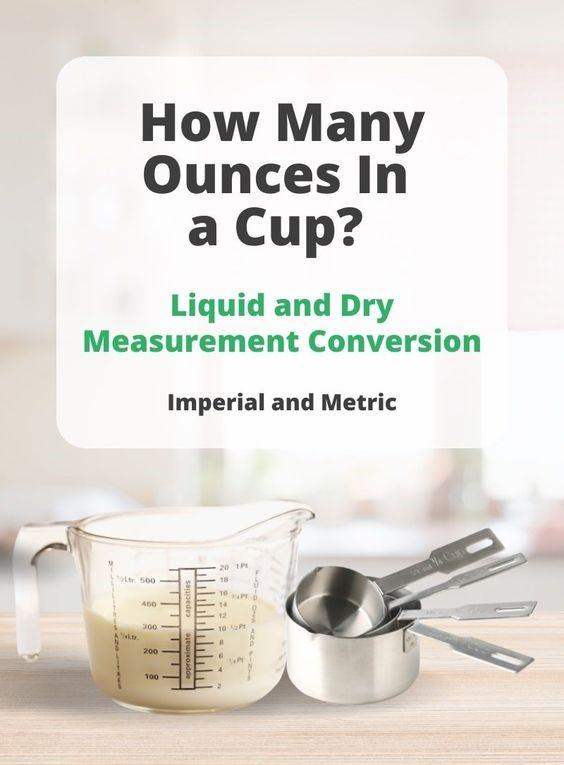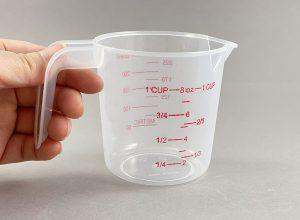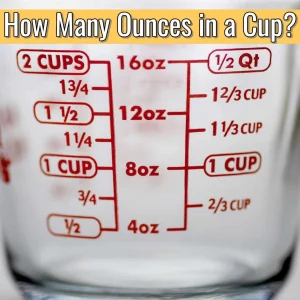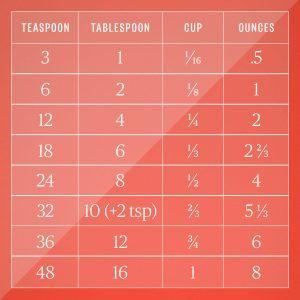When it comes to cooking and baking, precise measurements are critical to achieving the perfect taste and texture of your dish. One of the most commonly used measurements is the cup, but what if you need to convert it to ounces? In this article, we will provide you with a complete guide on how many ounces are in a cup and how to convert between the two units of measurement.

# Understanding Cups and Ounces

## What is a Cup?A cup is a unit of measurement used in cooking and baking. It is often used to measure liquid or dry ingredients, such as flour, sugar, or milk. One cup is equivalent to 8 fluid ounces or 16 tablespoons.

## What is an Ounce?

An ounce is a unit of weight measurement. It is commonly used to measure the weight of food items, such as meat or vegetables. One ounce is equivalent to 0.0625 pounds or 28.35 grams.

## How Many Ounces in a Cup?The number of ounces in a cup depends on the type of ingredient you are measuring. Here is a breakdown of how many ounces are in one cup for different ingredients:

• Liquid Ingredients: One cup of liquid is equal to 8 fluid ounces.
• Dry Ingredients: One cup of dry ingredients, such as flour or sugar, is equal to 4.5 to 5 ounces.
• Solid Ingredients: One cup of solid ingredients, such as butter or cheese, is equal to 8 ounces.

It is important to note that these measurements are based on the US customary system. Other countries may use different

measurements, so it is essential to double-check the unit of measurement before converting.

## How to Convert Cups to Ounces?

 1 cup of water 8 fluid ounces 1 cup of milk 8 fluid ounces 1 cup of flour 4.5 ounces 1 cup of sugar 7.1 ounces 1 cup of brown sugar 7.6 ounces 1 cup of butter 8 ounces

To convert the number of cups to ounces for an ingredient not listed above, you can use the following conversion factors:

 1 cup 8 fluid ounces 1 cup 4.2 ounces (dry ingredients)

Converting cups to ounces is a straightforward process. Here are the steps to convert cups to ounces:

1. Determine the type of ingredient you are measuring.
2. Find out how many ounces are in one cup for that ingredient.
3. Multiply the number of cups by the number of ounces in one cup.

For example, if you want to convert 2 cups of milk to ounces, you would follow these steps:

1. Determine that you are measuring a liquid ingredient.
2. Find out that one cup of liquid is equal to 8 fluid ounces.
3. Multiply 2 cups by 8 ounces to get the answer: 16 ounces.

## How to Convert Ounces to CupsConverting ounces to cups is also a simple process. Here are the steps to convert ounces to cups:

1. Determine the type of ingredient you are measuring.
2. Find out how many ounces are in one cup for that ingredient.
3. Divide the number of ounces by the number of ounces in one cup.

For example, if you want to convert 10 ounces of flour to cups, you would follow these steps:

1. Determine that you are measuring a dry ingredient.
2. Find out that one cup of flour is equal to 4.5 to 5 ounces.
3. Divide 10 ounces by 4.5 to 5 ounces to get the answer: approximately 2 cups.

## Tips for Accurate Measurement

Accurate measurement is crucial to achieving the desired results in your cooking and baking. Here are some tips to ensure your measurements are accurate:

1. Use a measuring cup and spoons instead of estimating the amount.
2. Level off dry ingredients, such as flour or sugar, with a straight edge to ensure an accurate measurement.
3. Use a kitchen scale to weigh solid ingredients, such as butter or cheese, for a precise measurement.